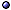Types of Actions for General Relativity

In General > s.a. gravitation; higher-order gravity theories.
* Possible contributions in four dimensions: Possible terms that can appear in the Lagrangian are [@ Zumino in(86)]

L0,4 = ea eb ec ed εabcd : Cosmological term ,
L1,2 = Rab ec ed εabcd : Einstein-Hilbert term ,
L2,0 = Rab Rcd εabcd : Euler invariant .

@ Related topics: Robinson IJTP(98) [chiral]; Mei a0711 [with positive kinetic-energy term]; Dubois-Violette & Lagraa LMP(09)-a0907 [large classes]; Banerjee & Majhi PRD(10) [and entropy]; Kolekar & Padmanabhan PRD(10)-a1005 [thermodynamic/holographic decomposition into surface and bulk terms]; Brown PRD(11)-a1008 [for the generalized harmonic formulation]; Kol & Smolkin PRD(12)-a1009 [in terms of Newtonian fields]; Demir et al a1105 [constructed solely from the Riemann tensor]; Sengupta JPCS(12) [with Nieh-Yan, Pontryagin and Euler topological terms].

Einstein-Hilbert Action > s.a. 2D gravity; noether charge.
* Expression: The variable is the metric (or its inverse); With a cosmological constant Λ, the volume term is

SEH[g] = $$1\over2\kappa$$M d4x |g|1/2 (R − 2Λ) ,

where $$\kappa = 8\pi G/c^4$$, which contains – linearly – second derivatives of g.
@ References: Hilbert KNGWG(15); Katanaev GRG(06)gq/05, TMP(06)gq [with |g| as a variable, polynomial]; Cheung & Remmen JHEP(17)-a1705 [as a theory of purely cubic interactions]; Gionti JPCS(19)-a1902 [Reuter-Weyer RG improved]; Takeuchi IJMPA(20)-a1811 [and Fisher information metric].

Gibbons-Hawking-York (tr K) Action (with all boundary terms) > s.a. extrinsic curvature.
* Idea: Obtained from the Hilbert-Einstein action by subtracting the boundary terms containing normal derivatives of the metric; To ensure that the induced metric is fixed on all components of ∂M, we must include all corner terms; For a four-dimensional M with initial and final hypersurfaces Σ1 and Σ2, and timelike boundary τ which meets Σi in Bi, i = 1, 2,

SGHY[g] = $$1\over2\kappa$${∫M d4v (R − 2Λ) + 2 [∫Σ2 (KK0) d3sΣ1 (KK0) d3sτ (KK0) d3sB2 ξ d2σ + B1 ξ d2σ] } ,

where K (K0) is the trace of the extrinsic curvature induced by g (g0) on ∂M, and ξ:= sinh−1(u · n), with u the future-pointing normal to Σ, and n the outward-pointing normal to τ.
@ References: Gibbons & Hawking PRD(77); York FP(86); Hayward PRD(93); Hawking & Hunter CQG(96)gq [boundaries]; Pons GRG(03)gq/01 [Lagrangian, Noether charges]; Polishchuk G&C(10) [and Hilbert-Einstein action].

3+1 Metric Form
* Expression: If θ = extrinsic curvature of τ; K extrinsic curvature of $$\Sigma_t$$; η = u · n; aa = ubb ua = acceleration of τ,

S[q, N, Na] = $$1\over2\kappa$$ dt Σ d3x N q1/2 (3R + Kab KabK2 − 2Λ) + $$1\over\kappa$$τ d3x |γ|1/2 (θ + ηKna aa) .

@ References: Hawking & Hunter CQG(96)gq.

Other Forms > s.a. action for general relativity [boundary terms].
* Baierlein-Sharp-Wheeler form: The one obtained when the Lagrange multiplier (the lapse function) is eliminated from the Lagrangian and one is left with a product of square roots.
@ General references: Ambjørn et al NPB(97)ht/96 [Regge-calculus inspired]; Arcioni et al JHEP(01)ht [boundary action, eikonal limit]; Cremaschini & Tessarotto EPJP(15)-a1609 [synchronous Lagrangian variational principles]; Cheung & Remmen JHEP(17)-a1612 [with twofold Lorentz symmetry]; Takeuchi a1811 [in terms of Fisher information metric]; Magnano et al a1812 [dependence on the Weyl tensor]; Schmekel a2009 [action for pure gravity as a boundary term].
@ BSW and related forms: Carlini & Greensite PRD(95)gq; Ó Murchadha IJMPA(02)-proc; Shyam & Venkatesh GRG(13)-a1209 [Barbour-Foster-Ó Murchadha 3-space action].
@ Curvature-saturated: Kleinert & Schmidt GRG(02)gq/00 [$$\cal L$$cs = $$\cal L$$EH/ (1 + l 4 R2)1/2, with l a length parameter].
@ Self-dual: Nieto & Socorro PRD(99)ht/98 [and Yang-Mills, MacDowell-Mansouri formalism]; Nieto MPLA(05)ht/04 [various versions]; > s.a. connection formulation.
@ Bimetric reformulation: Koivisto PRD(11)-a1103; Jiménez et al PRD(12)-a1201 [bimetric variational principle].Other forms: see action [effective classical action, similar theories]; first-order forms; formulations of general relativity [including embedding variables].